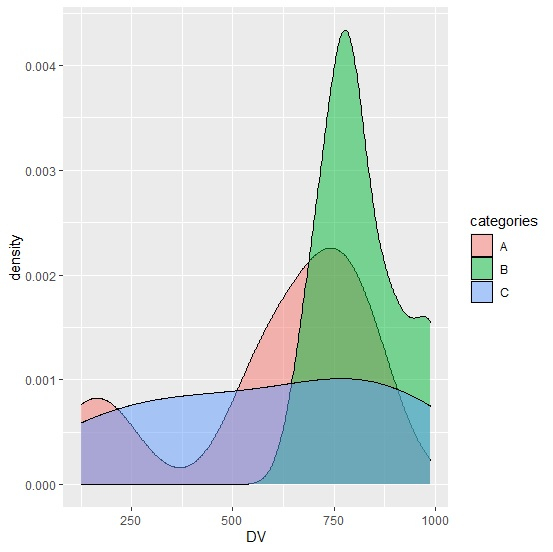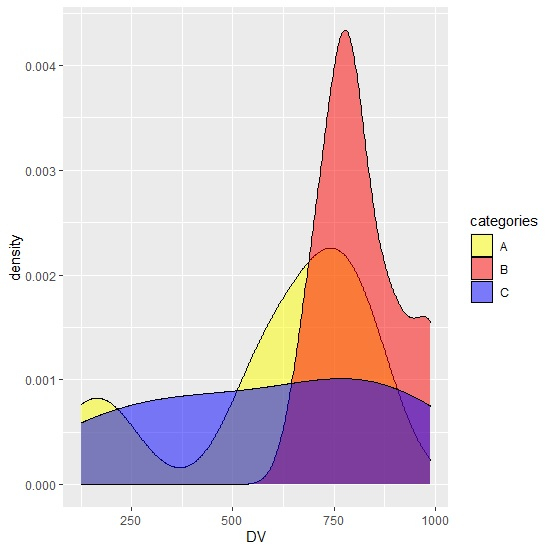# How to manually set the colors of density plot for categories in R?

To create density plot with manually setting the colors of categories, we can follow the below steps −

• Frist of all, create a data frame.
• Load ggplot2 package and creating the density plot for the categories.
• Create the density plot for the categories in the data frame by using scale_fill_manual function.

## Create the data frame

Let's create a data frame as shown below −

Live Demo

categories<-sample(LETTERS[1:3],20,replace=TRUE)
DV<-sample(1:1000,20)
df<-data.frame(categories,DV)
df

On executing, the above script generates the below output(this output will vary on your system due to randomization) −

  categories DV
1  A         681
2  C         770
3  A         204
4  B         692
5  A         852
6  A         125
7  B         797
8  C         464
9  A         769
10 C         923
11 C         184
12 A         816
13 A         755
14 A         554
15 A         577
16 B         989
17 A         683
18 B         887
19 B         787
20 B       757

## Load ggplot2 package and create density plot for categories

Use geom_density with fill function inside aes to create density plot for categories filled with different colors −

library(ggplot2)
categories<-sample(LETTERS[1:3],20,replace=TRUE)
DV<-sample(1:1000,20)
df<-data.frame(categories,DV)
ggplot(df,aes(DV))+geom_density(aes(fill=categories),alpha=0.5)

### Output## Create the density plot for categories by manually setting the colors

Use scale_fill_manual function of ggplot2 package to create the density plot with manually defining the colors of densities −

categories<-sample(LETTERS[1:3],20,replace=TRUE)
DV<-sample(1:1000,20)
df<-data.frame(categories,DV)
library(ggplot2)
ggplot(df,aes(DV))+geom_density(aes(fill=categories),alpha=0.5)+scale_fill_manual(val
ues=c("A"="yellow","B"="red","C"="blue"))

### Output